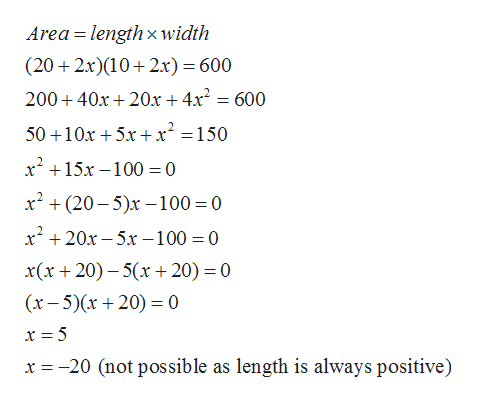# A pool measuring 10 meters by 20 meters is surrounded by a path of uniform width, as shown in the figure. If the area of the pool and the path combined is 600 square meters, what is the with of the path?

Question
120 views

A pool measuring 10 meters by 20 meters is surrounded by a path of uniform width, as shown in the figure. If the area of the pool and the path combined is 600 square meters, what is the with of the path?

check_circle

star
star
star
star
star
1 Rating
Step 1

Given a pool measuring 10 meters by 20 meters is surrounded by a path of uniform width and area of the pool and the path combined is 600 square meters. The figure is given below

Step 2

Now the area of the pool and the ...help_outlineImage TranscriptioncloseArea lengthx width (20 2x)(10 2x) = 600 20040x 20x + 4x2 = 600 5010x5x+ x2 = 150 x15x-100 0 х* + (20-5)х — 100- 0 х* + 20х - 5х — 100 %3D0 x(x+20)-5x 20) 0 (x-5)x+20) 0 x -20 (not possible as length is always positive) fullscreen

### Want to see the full answer?

See Solution

#### Want to see this answer and more?

Solutions are written by subject experts who are available 24/7. Questions are typically answered within 1 hour.*

See Solution
*Response times may vary by subject and question.
Tagged in
MathAlgebra

### Other